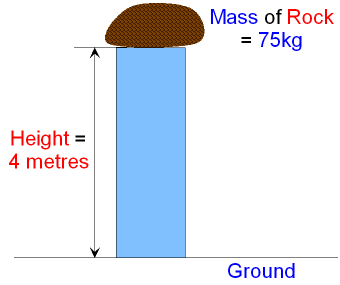gcsescience.com                                       26                                       gcsescience.com

Energy Transfer

Potential Energy can be Elastic or Gravitational.

What is Gravitational Potential Energy?

Gravitational Potential Energy called "GPE" is a
measure of how far an object can fall. The higher up
it is, the further it can fall and the more GPE it has.
GPE also depends on the object's weight.

GPE = mass x gravity x height.     GPE = m x g x h.

This equation is important!

Since mass x gravity = weightGPE = weight x height.
On Earth the force of gravity is 10 N/kg.

For example, the GPE of a rock that has a mass of 75kg
and is raised to a height of 4·0 metres is

GPE  =  75 x 10 x 4·0
= 3000J.When the object starts to fall, GPE is transferred into KE.

gcsescience.com          Physics Quiz          Index          Energy Quiz          gcsescience.com